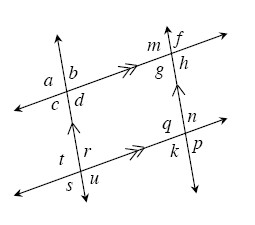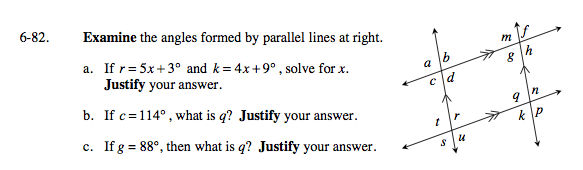### Home > GC > Chapter 6 > Lesson 6.2.5 > Problem6-82

6-82.
1.the angles formed by parallel lines at right. Homework Help ✎

1. If r = 5x + 3° and k = 4x + 9°, solve for x. Justify your answer.

3. If g = 88°, then what is q? Justify your answer.How are r and k related? How can you use this relationship to solve for x?

x = 6°

Does c relate to another angle that might help you determine the measure of q?

Relate c, t, r, or g.

What is the relationship between g and q? How does this help you determine the measure of q?